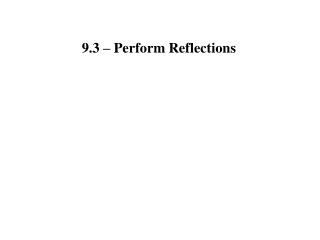DownloadDownload Presentation9.3 – Perform Reflections

# 9.3 – Perform Reflections

Download Presentation## 9.3 – Perform Reflections

- - - - - - - - - - - - - - - - - - - - - - - - - - - E N D - - - - - - - - - - - - - - - - - - - - - - - - - - -
##### Presentation Transcript

1. 9.3 – Perform Reflections

2. Reflection: Transformation that uses a line like a mirror to reflect an image Line of Reflection: Mirror line in a reflection

3. A reflection in a line m maps every point P in the plane to a point , such that: • If P is not on m, then m is the perpendicular bisector of • If P is on m, then

4. Reflect point P(5, 7)in the given line. x – axis P(5, 7) becomes P A reflection in the x-axis changes (x, y) into _______ (x, –y)

5. Reflect point P(5, 7)in the given line. y – axis P(5, 7) becomes P A reflection in the y-axis changes (x, y) into _______ (–x, y)

6. Reflect point P(5, 7)in the given line. y = x P(5, 7) becomes P A reflection in the y = x changes (x, y) into _______ (y, x)

7. Graph the reflection of the polygon in the given line. y = x (–1 , –3) (–3, –1) (2, –4 ) (–4, 2) (3, 0) (0, 3)

8. 9.4 – Perform Rotations

9. Transformation that turns a figure about a fixed point Rotation: Center of Rotation: The point that the rotation happens around Angle of Rotation: Degree the figure is rotated counterclockwise

10. A rotation about a point P through an angle of x° maps every point Q in the plane to a point such that: • If Q is not the center of rotation, then • and Q x° P

11. A rotation about a point P through an angle of x° maps every point Q in the plane to a point such that: • If Q is the center of rotation, then Q

12. State if the rotation is 90°, 180°, or 270° counter-clockwise. 180° Counter-clockwise degree of rotation: _______

13. State if the rotation is 90°, 180°, or 270° counter-clockwise. 90° Counter-clockwise degree of rotation: _______

14. State if the rotation is 90°, 180°, or 270° counter-clockwise. 270° Counter-clockwise degree of rotation: _______

15. 6.Find the value of each variable in the rotation. 4 x = z = 3 y = z + 2 y = 3 + 2 y = 5

16. 6.Find the value of each variable in the rotation. 4s = 24 s = 6 r = 2s – 3 r = 2(6) – 3 r = 12 – 3 r = 9

17. HW Problems 9.3 #7